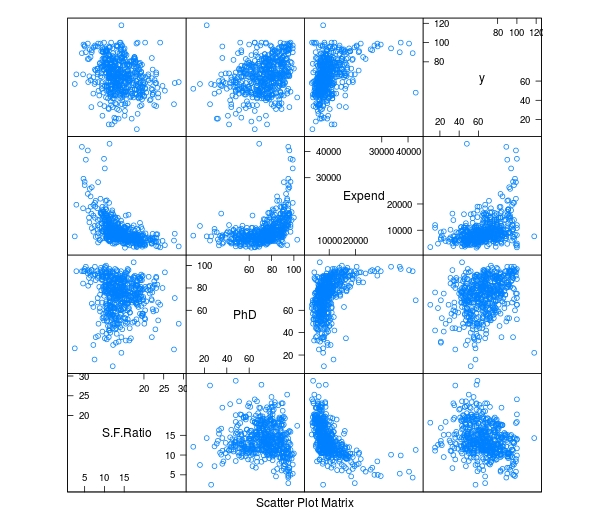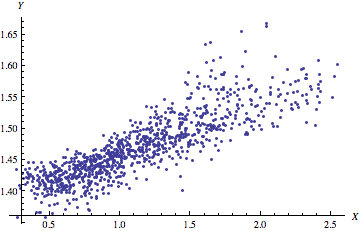# Multiple Regression Prediction in R

In this post, we will learn how to predict using multiple regression in R. In a previous post, welearn how to predict with simple regression. This post will be a large repeat of this other post with the addition of using more than one predictor variable. We will use the “College” dataset and we will try to predict Graduation rate with the following variables

• Student to faculty ratio
• Percentage of faculty with PhD
• Expenditures per student

Preparing the Data

First we need to load several packages and divide the dataset int training and testing sets. This is not new for this blog. Below is the code for this.

```library(ISLR); library(ggplot2); library(caret)
data("College")
p=0.7, list=FALSE)
trainingset <- College[inTrain, ]
testingset <- College[-inTrain, ]
dim(trainingset); dim(testingset)```

Visualizing the Data

We now need to get a visual idea of the data. Since we are using several variables the code for this is slightly different so we can look at several charts at the same time. Below is the code followed by the plots

```> featurePlot(x=trainingset[,c("S.F.Ratio","PhD","Expend")],y=trainingset\$Grad.Rate, plot="pairs")```

To make these plots we did the following

1. We used the ‘featureplot’ function told R to use the ‘trainingset’ data set and subsetted the data to use the three independent variables.
2. Next, we told R what the y= variable was and told R to plot the data in pairs

Developing the Model

We will now develop the model. Below is the code for creating the model. How to interpret this information is in another post.

`> TrainingModel <-lm(Grad.Rate ~ S.F.Ratio+PhD+Expend, data=trainingset) > summary(TrainingModel)`

As you look at the summary, you can see that all of our variables are significant and that the current model explains 18% of the variance of graduation rate.

Visualizing the Multiple Regression Model

We cannot use a regular plot because are model involves more than two dimensions.  To get around this problem to see are modeling, we will graph fitted values against the residual values. Fitted values are the predict values while residual values are the acutal values from the data. Below is the code followed by the plot.

```> CheckModel<-train(Grad.Rate~S.F.Ratio+PhD+Expend, method="lm", data=trainingset)
> DoubleCheckModel<-CheckModel\$finalModel
> plot(DoubleCheckModel, 1, pch=19, cex=0.5)```
``````

Here is what happened

1. We created the variable ‘CheckModel’.  In this variable, we used the ‘train’ function to create a linear model with all of our variables
2. We then created the variable ‘DoubleCheckModel’ which includes the information from ‘CheckModel’ plus the new column of ‘finalModel’
3. Lastly, we plot ‘DoubleCheckModel’

The regression line was automatically added for us. As you can see, the model does not predict much but shows some linearity.

Predict with Model

We will now do one prediction. We want to know the graduation rate when we have the following information

• Student-to-faculty ratio = 33
• Phd percent = 76
• Expenditures per Student = 11000

Here is the code with the answer

```> newdata<-data.frame(S.F.Ratio=33, PhD=76, Expend=11000)
> predict(TrainingModel, newdata)
1
57.04367```

To put it simply, if the student-to-faculty ratio is 33, the percentage of PhD faculty is 76%, and the expenditures per student is 11,000, we can expect 57% of the students to graduate.

Testing

We will now test our model with the testing dataset. We will calculate the RMSE. Below is the code for creating the testing model followed by the codes for calculating each RMSE.

```> TestingModel<-lm(Grad.Rate~S.F.Ratio+PhD+Expend, data=testingset)
```
```> sqrt(sum((TrainingModel\$fitted-trainingset\$Grad.Rate)^2))
 369.4451
 219.4796```

Here is what happened

1. We created the ‘TestingModel’ by using the same model as before but using the ‘testingset’ instead of the ‘trainingset’.
2. The next two lines of codes should look familiar.
3. From this output the performance of the model improvement on the testing set since the RMSE is lower than compared to the training results.

Conclusion

This post attempted to explain how to predict and assess models with multiple variables. Although complex for some, prediction is a valuable statistical tool in many situations.# Correlation

A correlation is a statistical method used to determine if a relationship exists between variables.  If there is a relationship between the variables itindicates a departure from independence. In other words, the higher the correlation the stronger the relationship and thus the more the variables have in common at least on the surface.

There are four common types of relationships between variables there are the following

1. positive-Both variables increase or decrease in value
2. Negative- One variable decreases in value while another increases.
3. Non-linear-Both variables move together for a time then one decreases while the other continues to increase
4. Zero-No relationship

The most common way to measure the correlation between variables is the Pearson product-moment correlation aka correlation coefficient aka r.  Correlations are usually measured on a standardized scale that ranges from -1 to +1. The value of the number, whether positive or negative, indicates the strength of the relationship.

The Person Product Moment Correlation test confirms if the r is statistically significant or if such a relationship would exist in the population and not just the sample. Below are the assumptions

• Subjects are randomly selected
• Both populations are normally distributed

Here is the process for finding the r.

1. Determine hypotheses
• H0: = 0 (There is no relationship between the variables in the population)
• H0: r ≠ 0 (There is a relationship between the variables in the population)
2. Decided what the level of significance will be
3. Calculate degrees of freedom to determine the t critical value (computer does this)
4. Calculate Pearson’s (computer does this)
5. Calculate t value (computer does this)
6. State conclusion.

Below is an example

A clerk wants to see if there is a correlation between the overall grade students get on an exam and the number of words they wrote for their essay. Below are the results

1                             79                           147
2                             76                           143
3                             78                           147
4                             84                           168
5                             90                           206
6                             83                           155
7                             93                           192
8                             94                           211
9                             97                           209
10                          85                           187
11                          88                           200
12                          82                           150

Step 1: State Hypotheses
H0: There is no relationship between grade and the number of words on the essay
H1: There is a relationship between grade and the number of words on the essay

Step 2: Level of significance
Set to 0.05

Step 3: Determine degrees of freedom and t critical value
t-critical = + 2.228 (This info is found in a chart in the back of most stat books)

Step 4: Compute r
r = 0.93                       (calculated by the computer)

Step 5: Decision rule. Calculate t-value for the r

t-value for r = 8.00  (Computer found this)

Since the computed t-value of 8.00 is greater than the t-critical value of 2.228 we reject the null hypothesis.

Step 6: Conclusion
Since the null hypothesis was rejected, we conclude that there is evidence that a strong relationship between the overall grade on the exam and the number of words written for the essay. To make this practical, the teacher could tell the students to write longer essays if they want a better score on the test.

IMPORTANT NOTE

When a null hypothesis is rejected there are several possible relationships between the variables.

• Direct cause and effect
• The relationship between X and Y may be due to the influence of a third variable not in the model
• This could be a chance relationship. For example, foot size and vocabulary. Older people have bigger feet and also a larger vocabulary. Thus it is a nonsense relationship

# Curriculum Design: Correlation Design

Correlation design is similar to broad-field design in that it is focused on integration. The difference is that correlation design combines only two subjects while broad-field will combine several subjects.  In many ways, one could say that correlation design is a simplistic version of broad-field design.Some examples of correlation design include biochemistry, which is the combining of biology and chemistry. Other examples include social psychology, which is sociology and psychology; bio-statistics, which is biology and statistics; and music technology, which focuses on music and its use through technology.  Generally, correlation design is found at the university level where students need expertise in specific subjects.

The advantages of correlation design are that it fills in the gaps within curriculum of two subjects that are related. The two subjects are combined in innovative ways and the students are able to see the connections between the two of them.

The disadvantages are that few teachers have enough expertise in the two subjects to successful correlate them in a curriculum. In addition, few teachers have the time to collaborate with their peers on a project such as this.  Despite these issues, correlation design is an option for teachers interested in creating a unique curriculum for the needs of their students.